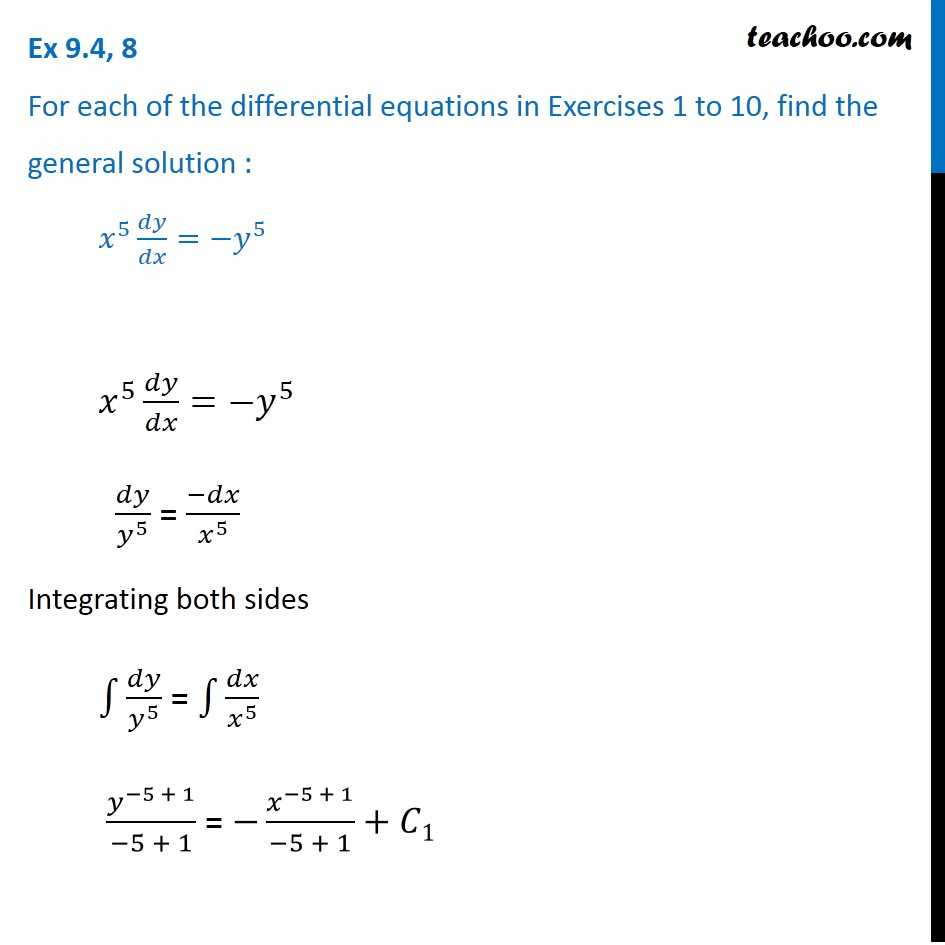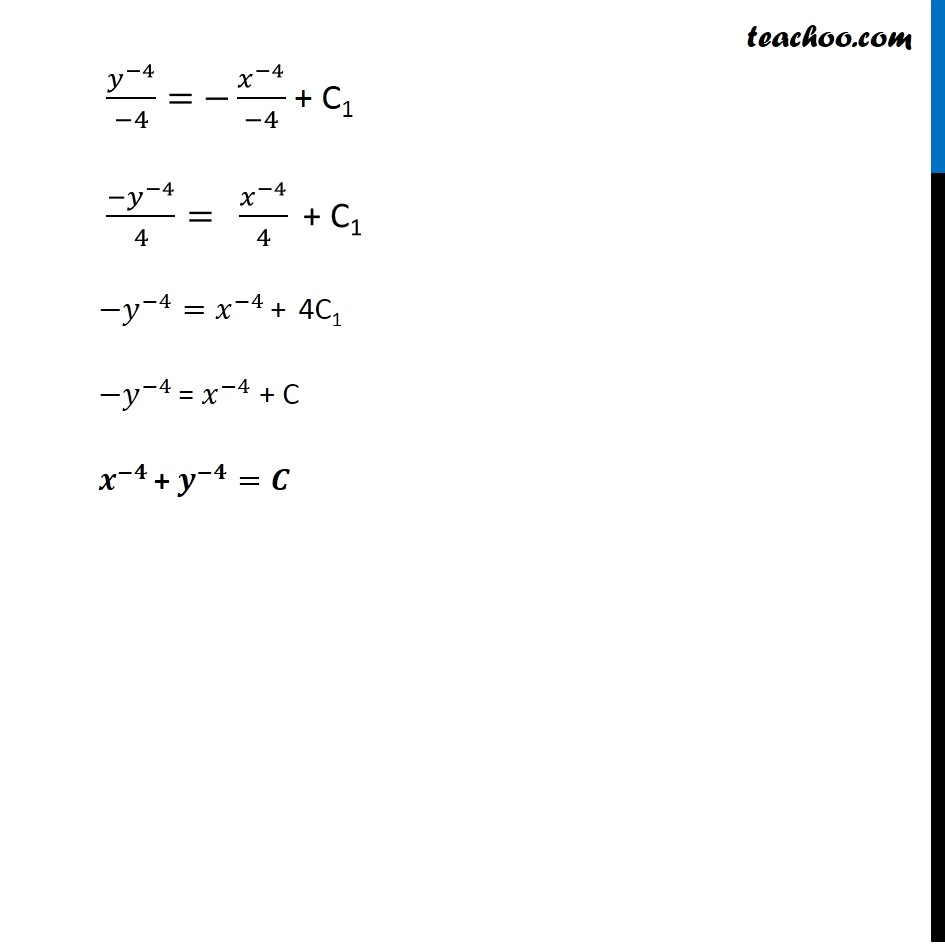Ex 9.4

Chapter 9 Class 12 Differential Equations
Serial order wiseGet live Maths 1-on-1 Classs - Class 6 to 12

### Transcript

Ex 9.4, 8 For each of the differential equations in Exercises 1 to 10, find the general solution : 𝑥^5 𝑑𝑦/𝑑𝑥=−𝑦^5 𝑥^5 𝑑𝑦/𝑑𝑥=−𝑦^5 𝑑𝑦/𝑦^5 = (−𝑑𝑥)/𝑥^5 Integrating both sides ∫1▒𝑑𝑦/𝑦^5 = ∫1▒𝑑𝑥/𝑥^5 𝑦^(−5 + 1)/(−5 + 1) = −𝑥^(−5 + 1)/(−5 + 1)+𝐶1 𝑦^(−4)/(−4)= −𝑥^(−4)/(−4) + C1 〖−𝑦〗^(−4)/4=" " 𝑥^(−4)/4 " + C1" −𝑦^(−4)=𝑥^(−4) + 4C1 −𝑦^(−4) = 𝑥^(−4) + C 𝒙^(−𝟒) + 𝒚^(−𝟒)=𝑪